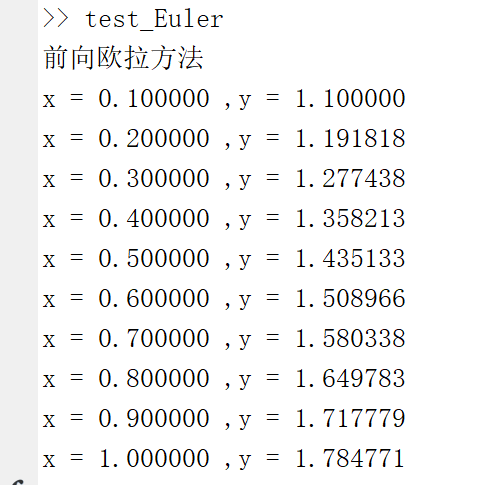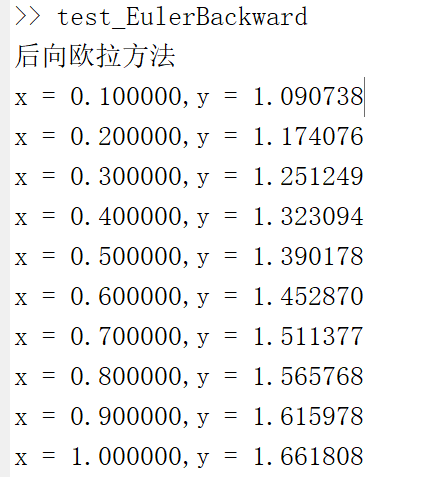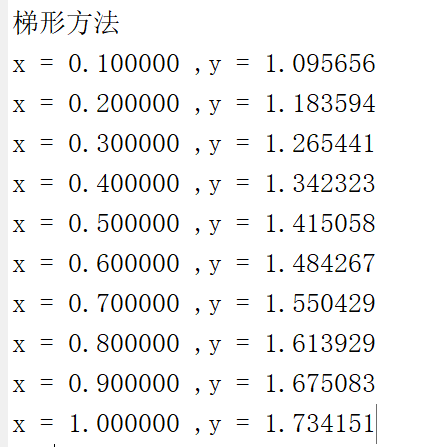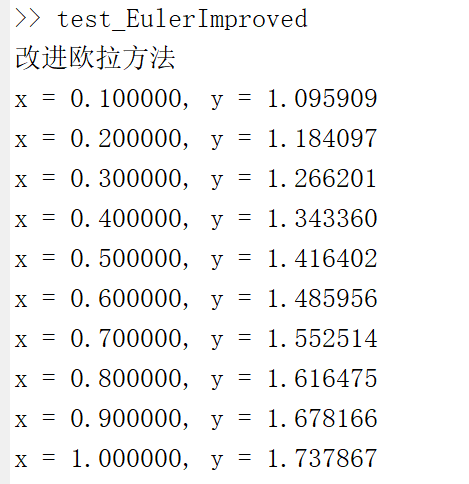# Halo

A magic place for coding

0%

## 介绍

本题涉及到的是常微分方程初值问题的求解方法。求解常微分方程可以分为两类解法：（1）解析方法；（2）数值解法。前者仅限于对特殊的方程进行求解，而后者可以应用于一般方程的求解。
常微分方程初值问题的一般形式是：
$$y^{‘} = f(x,y), x \in [x_0, b],\y(x_0) = y_0，$$

## 分析

### 1.前向欧拉法

在$xy$平面上，微分方程$f(x,y) = y^{‘}$的解$y=y(x)$称作它的积分曲线。从几何角度出发，从初始点$P_0(x_0,y_0)$出发，沿该点切线方向$f(x_0,y_0)$推进到$x=x_1$上的一点$P_1$，然后再从$P1$点推进到$x=x_2$上的一点$P_2$，一直推进到$P_n$。
对于相邻的两个点$P_n$和$P_{n+1}$，有如下关系：
$$\frac{y_{n+1} - y_n}{x_{n+1} - x_n} = f(x_n,y_n)，$$

$$y_{n+1} = y_n + hf(x_n,y_n)，$$

### 2.后向欧拉法

如果对微分方程从$x_0$到$x_{n+1}$积分，可以得到：
$$y(x_{n+1}) = y(x_n) + \int^{x_{n+1}}{x_n}f(t,y(t))dt，$$

$$y_{n+1} = y_n + hf(x_{n+1},y {n+1})，$$

隐式方法计算的时候，要使用迭代法，逐步显式化。首先使用欧拉公式提供初值，再进行迭代。
$$y^{(0)}{n+1} = y_n + hf(x_n,y_n)\ y^{(k+1)}_{n+1} = y_n + hf(x_{n+1},y^{(1)} {n+1})$$

### 3.梯形方法

在欧拉方法的基础上，对积分右端近似的方法进行改进，使用梯形求积公式近似，可以得到精度更高的计算公式，主要形式为：
$$y_{n+1} = y_n + \frac{h}{2}[f(x_n,y_n) + f(x_{n+1}, y_{n+1})]，$$

$$y^{(0)}{n+1} = y_n + hf(x_n,y_n) \ y^{(k+1)}_{n+1} = y_n + \frac{h}{2}[f(x_n,y_n) + f(x_{n+1},y^{(k)} {n+1})], k = 0,1,2,\dots$$

### 4.改进欧拉方法

梯形方法给我们提供了一种获得更高精度的算法，但是其计算量是庞大的，因为每步迭代都需要重新计算$f(x,y)$的值。这里给出了一种思路：先使用欧拉方法求得一个初步的近似值$\overline y_{n+1}$,\，称为预测值，但是这个预测值的精度可能会很差，因此需要校正。这里使用梯形公式对其进行校正，得到一个校正值$y_{n+1}$。这种预测-校正系统称为改进的欧拉公式
$$\begin{cases} 预测 \overline y_{n+1} = y_n + hf(x_n,y_n)，\ 校正 y_{n+1} = y_n + \frac{h}{2}[f(x_n,y_n) + f(x_{n+1}, \overline y_{n+1})] \end{cases}$$

## 代码实现

### 1.前向欧拉法

根据上述推导的公式，编写matlab代码：

### 2.后向欧拉法

根据上述推导的公式，编写matlab代码：

### 3.梯形方法

根据上述推导的公式，编写matlab代码：

### 4.改进欧拉方法

根据上述推导的公式，编写matlab代码：

## 小结由上述几种方法可以看出，前向欧拉方法和后向欧拉方法的误差是比较大的，改进的欧拉方法精度比欧拉法要好，而在四种方法中，梯形方法拥有更高的精度。但是从计算复杂度而言，欧拉法是比较简单的。考虑到数值稳定性的问题，有时可以使用后向欧拉方法来保证数值的稳定性。综合计算复杂度和精度而言，个人认为改进欧拉方法是一个比较好的折中方案

#### 参考资料：

1.数值分析（第5版） 李庆扬，王能超，易大义

Welcome to my other publishing channels